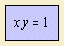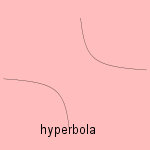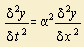# hyperbola

## conic sectionThe hyper part in the hyperbola word is derived from the Greek word 'huper', what means to surpass a certain limit 1). This excess is reached while adapting the areas in the old Greek way, in the case of the hyperbola. The same quality we find in the hyperbole, what has the meaning of a stylistic exaggeration. For example: an ocean of tears. Apollonius gave the hyperbola its name. Nowadays a more common definition of the hyperbola is as being the curve for which the difference between the distances to the foci remains constant. Pierre de Fermat wrote in 1636 in a letter to Roberval that he had found the generalization of the curve, that has later been given the name of hyperbola of Fermat, 7 years earlier. The curve can also be written as x2 - y2 = 1 or r2cos2φ=1. The hyperbola has two asymptotes, what can be used to describe saturation-growth2). Three special cases can be distinguished: orthogonal hyperbola, equilateral hyperbola.or rectangular hyperbola: the two asymptotes are perpendicular. This special case has been studied at first by the Greek Menaechmos. degenerate hyperbola: the hyperbola has been degenerated to his asymptotes  adjungated hyperbola: given a hyperbola a second one is added, with the same asymptotes, but axes switched. An example of this relationship is formed by a pair of hyperbolas x y = 1 or x y = -1 Some relations with other curves: the hyperbola is the isoptic of the parabola the hyperbola can be seen as a sinusoidal spiral depending on the center of inversion and the form of the hyperbola, as polar inverse the following curves can be obtained: lemniscate (of Bernoulli) (center) (right) strophoid (vertex) trisectrix of MacLaurin (vertex) the pedal curve (focus) is the circle a pedal (center) of the rectangular hyperbola is the lemniscate its isoptic is the circle The Greek Euklid and Aristaeus wrote about the general hyperbola, although they confined the curve to one branch. Apollonius was the first to see both of the two branches. Where do we see the hyperbola in our world? Well, take a cistern, filled with water, and place two oscillators, with same frequency and amplitude. Then you see an extinction in the form of hyperbolas, as result of the constant difference in distance between the two foci (oscillators), on a hyperbola. Above that, we find the hyperbola in various branches of science as variables, that are inversely proportional related. It appears that the attention for the recent past is inversely proportional to the passed time3). The American linguist George Kingsley Zipf counted articles' word occurrences. When he arranged the words in order of frequency, the product of number and frequency turned out to be about a constant. In other words: the most used word occurs a 10 ten times more often than the word on the 10th place. Zipf studied a lot of texts, differing in languages and subject, but once again he found the amazing relation. Later would have been proven that also texts with random grouped elements (out an alphabet plus the space) obey the rule4). George Kingsley got more and more enthusiastic and he discovered similar relations on other subjects, as in the distribution of inhabitants, valid in different countries. The relation is called a hyperbolic distribution, and in the case of number and frequency the relations was named the Zipf's law. Zipf believed in the validity of his law, but so hard that he justified the annexation of Austria by Germany by stating that his law would fit better! After Zipf's first investigations the relation has been found on many subjects: from the distribution of the reptiles sorts in families to the number of articles handling different topics in a scientific magazine. And recently biologists found that (non coding) junk DNA obeys Zipf's law, but the coding DNA does not. Sometimes a distribution can not be matched with a hyperbola, the Mandelbrot-Zipf law makes a generalization towards the power function: y = x -a 5) In another math region we can find the hyperbolic geometry (or geometry of Lobachevsky), one of the non-Euclidean geometries, based on the axiom that there exist at least two lines through a point P that are parallel to a line not through P. The hyperbolic functions have not much in common with the hyperbola. Their name is based on the fact that two hyperbolic functions can be drawn geometrically in a hyperbola. The wave equation, a partial differential equation of second order, is also named the hyperbolic differential equation. Reason is the resemblance between the equations  6). The hyperbolism of a curve f (x,y) = 0 is a curve with equation f (x, xy) = 0. notes 1) Bole is from balloo (Gr.) = to throw 2) In the form: y = x / (1 + x) 3) Law of the extinguishing past, based on the frequency of year numbers in newspapers: Pollmann 1996 4) Maybe this is true, but i can't get to this law (for an one or two letter alphabet). 5) For the cities and their inhabitants in many cases a value of the constant a between 0 and 1 has been found. Australia is an exception with a value of a of about 1.2. 6) Substitute the partial derivatives by x en y in: